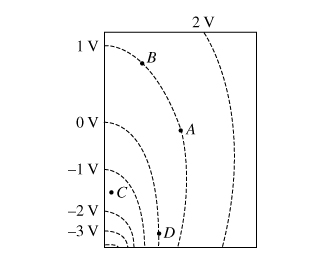# Problem: The dashed lines in the diagram represent cross sections of equipotential surfaces drawn in 1-V increments.The magnitude of the electric field at point C isa) greater than the magnitude of the electric field at point B.b) less than the magnitude of the electric field at point B.c) equal to the magnitude of the electric field at point B.d) unknown because the value of the electric potential at point C is unknown.

###### FREE Expert Solution

The electric field is constant over equipotential surface.

Also, the electric field strength is inversely proportional to the spacing between the equipotential surfaces.

82% (310 ratings)###### Problem Details

The dashed lines in the diagram represent cross sections of equipotential surfaces drawn in 1-V increments.The magnitude of the electric field at point C is

a) greater than the magnitude of the electric field at point B.

b) less than the magnitude of the electric field at point B.

c) equal to the magnitude of the electric field at point B.

d) unknown because the value of the electric potential at point C is unknown.